• 模拟 2 个已知信号的带通滤波器（它不适用于随机信号）。 - 将信号转换为频域。 - 去除不需要的频率（即 6.5 Hz）。 - 再次将信号转换为时域。 所有模拟都在没有内置傅立叶传输的情况下完成。 感谢 Mike X Cohen ...matlab
• 对信道带通滤波器的输入输出的噪声的时域频域特性进行统计和分析，画出其时域和频域的图形。 高斯白噪声产生 首先确定采样频率和总时长，以此确定总采样点数和时间向量： fs=1000;%采样频率hz T_N=1;%总时间s t=1/...
通信原理大作业中的一部分，使用matlab仿真：
产生信道高斯白噪声，设计信道带通滤波器对高斯白噪声进行滤波，得到窄带高斯噪声。对信道带通滤波器的输入输出的噪声的时域、频域特性进行统计和分析，画出其时域和频域的图形。
高斯白噪声产生
首先确定采样频率和总时长，以此确定总采样点数和时间向量：
fs=1000;%采样频率hz
T_N=1;%总时间s
t=1/fs:1/fs:T_N;%时间向量
L=T_N*fs;%样本数量
然后用wgn产生高斯噪声：
z=wgn(L,1,power);
当然，也可以用原始的产生正态随机数的方法：
z=sigma.*randn(L,1)
注意power的单位是dbW，转换公式如下：

p

(

d

B

w

)

=

10

log

⁡

P

(

W

)

p (dBw) = 10\log P (W)

如果功率P为1w，折算为dBw后为0dBw。 另外高斯白噪声的方差是噪声功率：

P

=

σ

2

P=\sigma^2

下面到了关键的环节，快速（离散）傅里叶变换fft：
fft_z=fft(z);
对噪声z从时域转换为频域，注意到如果要获得单边频谱，还需要做以下操作：
P = abs(fft_z/L);%取幅频特性，除以L
P = P(1:L/2+1);%截取前半段
P(2:end-1)=2*P(2:end-1);%单侧频谱非直流分量记得乘以2
f = fs*(0:(L/2))/L;%频率，最多到一半（奈奎斯特采样定理）
fft的结果是关于采样频率的一半对称的，幅度需要除以采样点个数L。从双边谱到单边谱需要对非直流分量乘以2。注意到由于奈奎斯特采样定理，原信号的最大频率不会超过采样频率的一半。假如我们设置采样频率为1000Hz，那么频域的最大频率也就是500Hz。 这些操作都是从mathwork官网上找到的，参考链接。不得不说matlab的帮助文档尤其是里面的示例真香！
高斯白噪声的时域和频域图如下：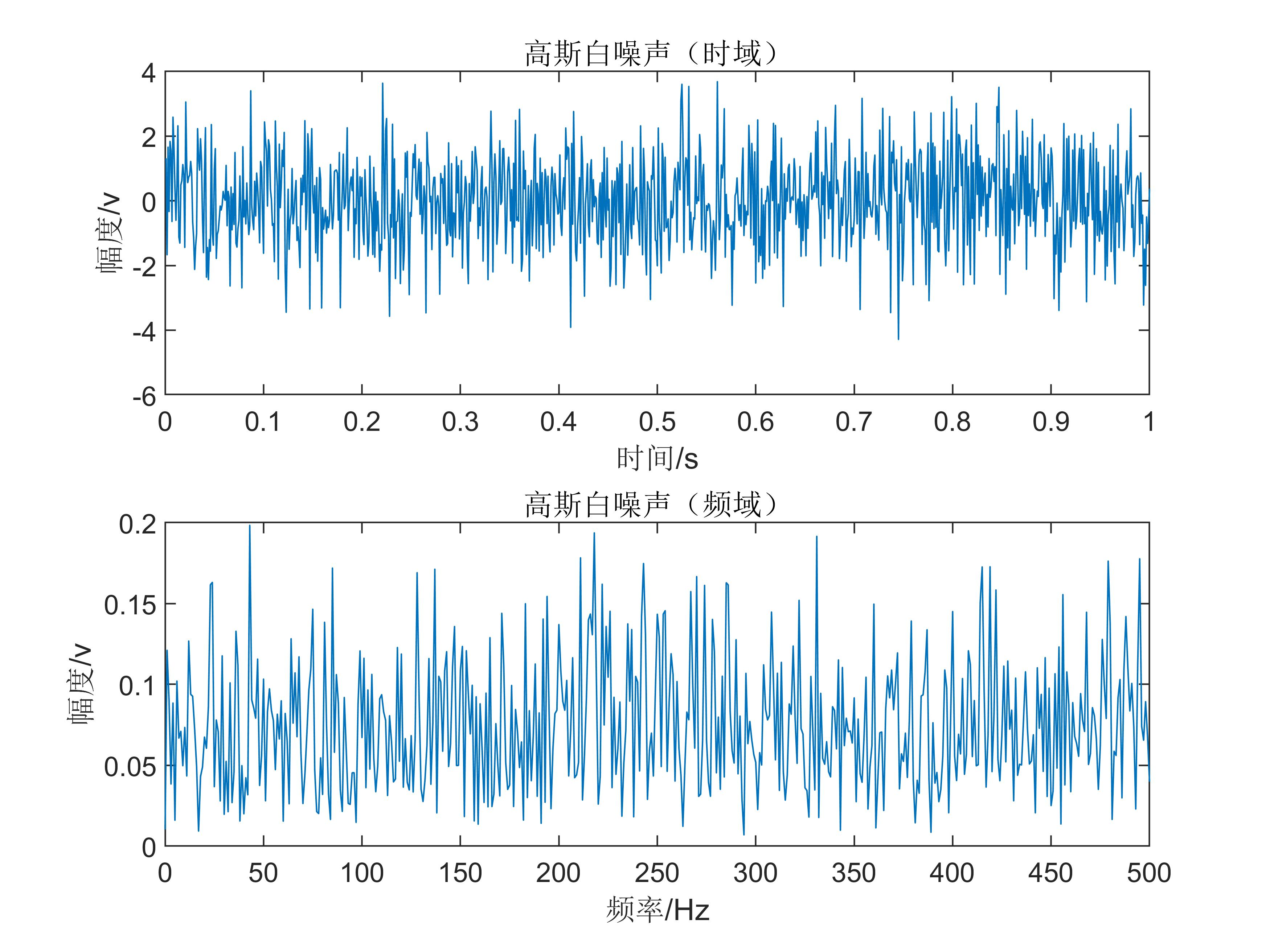白噪声白噪声，就是频谱上也是到处都是高斯分布。
巴特沃斯滤波器
用butter函数获得8阶巴特沃斯滤波器系数，带通范围100-200Hz
[b,a]=butter(8,[100/(fs/2),200/(fs/2) ]);
第一个参数是滤波器阶数，第二个参数是归一化的带通频率，注意到fs/2是信号的最大频率。 用下面这个函数可以画出滤波器特性曲线：
freqs(b,a)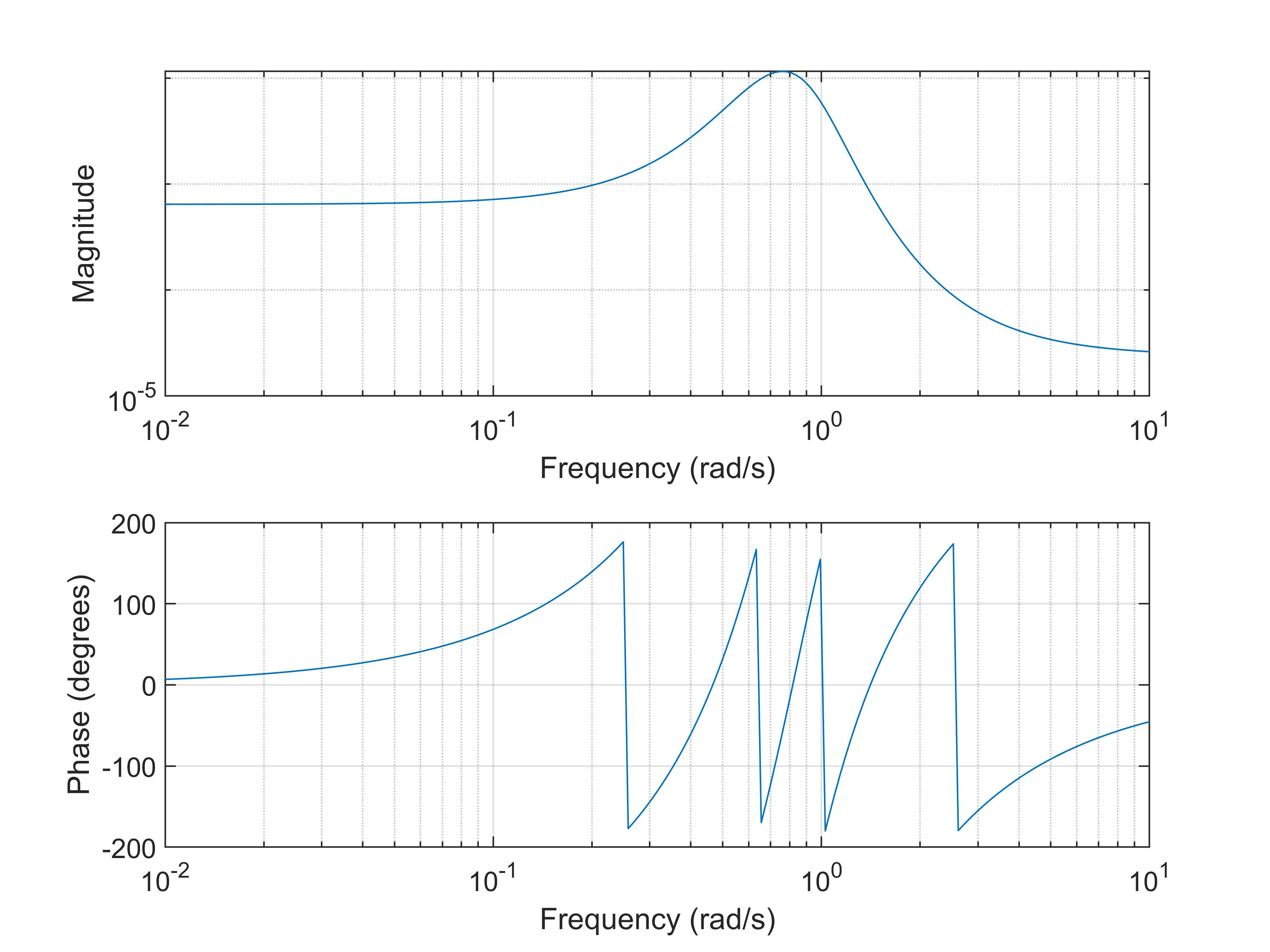（我其实看不懂）
滤波
用flutter函数快乐的滤波~
lvbo_z=filter(b,a,z);
应该是对原时域信号z滤波，我原先这里对fft后的信号滤波了，结果怎么都不对，debug了好久。 滤波后的信号lvbo_z也是个时域信号，用上述相同的办法fft后画单边频谱，可以得到窄带高斯噪声：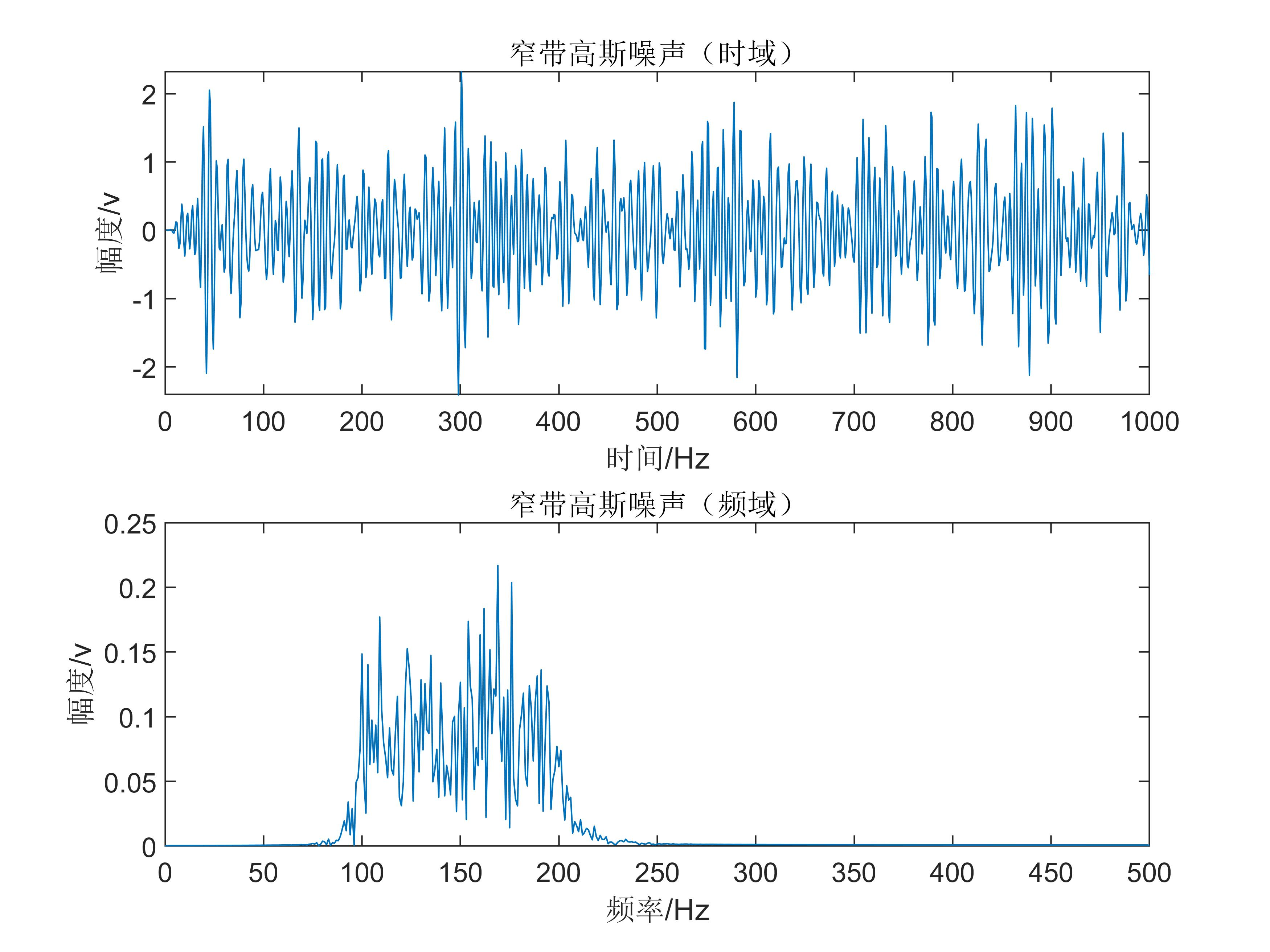可以明显发现滤波后的信号时域上看起来更奇怪了，频域上看，是把100-200Hz频率分量保留，其余频率分量滤除了。 做个对比，带通范围调到300-400Hz: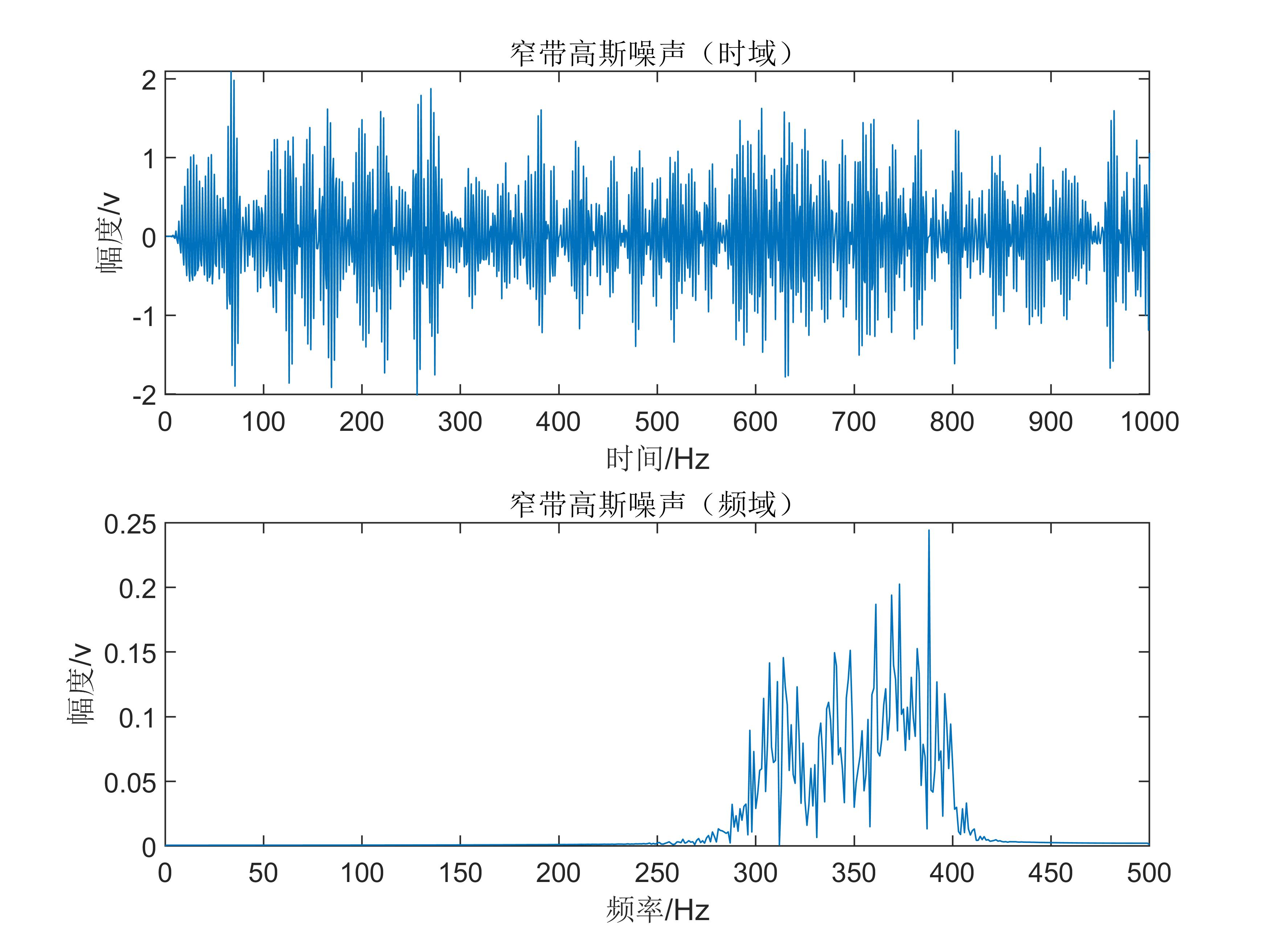频率更高了，波形看起来更密了。

刚刚学了怎么做动图，这就做了个放个对比图在这里：好家伙，制作动图都要花钱，不花钱就给你贴水印，这一点也不开源。

全部代码：
fs=1000;%采样频率hz
T_N=1;%总时间s
t=1/fs:1/fs:T_N;%时间向量
L=T_N*fs;%样本数量
power=3;%噪声功率,单位为dbw
z=wgn(L,1,power);
subplot(2,1,1)
plot(t,z)
xlabel("时间/s")
ylabel("幅度/v")
title("高斯白噪声（时域）")

fft_z=fft(z);%快速傅里叶变换之后的噪声
P = abs(fft_z/L);%取幅频特性，除以L
P = P(1:L/2+1);%截取前半段
P(2:end-1)=2*P(2:end-1);%单侧频谱非直流分量记得乘以2
f = fs*(0:(L/2))/L;%频率，最多到一半（奈奎斯特采样定理）
subplot(2,1,2)
plot(f,P)
xlabel("频率/Hz")
ylabel("幅度/v")
title("高斯白噪声（频域）")

[b,a]=butter(8,[300/(fs/2),400/(fs/2) ]);%获得8阶巴特沃斯滤波器系数，100-200Hz
figure(2)
freqs(b,a)%画滤波器特性曲线
lvbo_z=filter(b,a,z);%滤波

figure(3)
subplot(2,1,1)
plot((lvbo_z))
xlabel("时间/Hz")
ylabel("幅度/v")
title("窄带高斯噪声（时域）")

fft_lvbo_z=fft(lvbo_z);%傅里叶变换
P = abs(fft_lvbo_z/L);%取幅频特性，除以L
P = P(1:L/2+1);%截取前半段
P(2:end-1)=2*P(2:end-1);%单侧频谱非直流分量记得乘以2
subplot(2,1,2)
plot(f,P)
xlabel("频率/Hz")
ylabel("幅度/v")
title("窄带高斯噪声（频域）")
书上的知识，尤其是技术上的，网络上还真是难找啊。

谢邀，通信原理80，班里倒数。
展开全文• 基于MATLAB对带限信号进行滤波处理并作出时域图和频域的图像，对信号进行处理并分析和对比。
• hbchifan的回答： % 用切比雪夫最佳一致逼近设计线性相位FIR带通滤波器； %信号为0.5hz, 0.9hz, 1.1hz和1.5hz的正统信号叠加组成 %通带为[0.9,1.1] %频谱分辨率与信号实际长度N成正比 clear all; f1=0.5;f2=0.9;f3=...

盘国英谭婷的回答：
你自己整合吧，我没时间帮你整合，我给你提供一些程序：
绝对正确的代码：程序1：
fs=22050;
%语音信号采样频率为22050
critical
stop.wav');
%读取语音信号的数据，赋给变量x1
sound(x1,22050);
%播放语音信号
y1=fft(x1,1024);
%对信号做1024点fft变换
f=fs*(0:511)/1024;
figure(1)
plot(x1)
%做原始语音信号的时域图形
title('原始语音信号');
xlabel('time
n');
ylabel('fuzhi
n');
figure(2)
freqz(x1)
%绘制原始语音信号的频率响应图
title('频率响应图')
figure(3)
subplot(2,1,1);
plot(abs(y1(1:512)))
%做原始语音信号的fft频谱图
title('原始语音信号fft频谱')
subplot(2,1,2);
plot(f,abs(y1(1:512)));
title('原始语音信号频谱')
xlabel('hz');
ylabel('fuzhi');
程序2：
fs=22050;
%语音信号采样频率为22050
critical
stop.wav');
%读取语音信号的数据，赋给变量x1
t=0:1/22050:(size(x1)-1)/22050;
y1=fft(x1,1024);
%对信号做1024点fft变换
f=fs*(0:511)/1024;
x2=randn(1,length(x1));
%产生一与x长度一致的随机信号
sound(x2,22050);
figure(1)
plot(x2)
%做原始语音信号的时域图形
title('高斯随机噪声');
xlabel('time
n');
ylabel('fuzhi
n');
randn('state',0);
m=randn(size(x1));
x2=0.1*m+x1;
sound(x2,22050);%播放加噪声后的语音信号
y2=fft(x2,1024);
figure(2)
plot(t,x2)
title('加噪后的语音信号');
xlabel('time
n');
ylabel('fuzhi
n');
figure(3)
subplot(2,1,1);
plot(f,abs(y2(1:512)));
title('原始语音信号频谱');
xlabel('hz');
ylabel('fuzhi');
subplot(2,1,2);
plot(f,abs(y2(1:512)));
title('加噪后的语音信号频谱');
xlabel('hz');
ylabel('fuzhi');
根据以上代码，你可以修改下面有错误的代码
程序3:双线性变换法设计butterworth滤波器
fs=22050;
t=0:1/22050:(size(x1)-1)/22050;
au=0.03;
d=[au*cos(2*pi*5000*t)]';
x2=x1+d;
wp=0.25*pi;
ws=0.3*pi;
rp=1;
rs=15;
fs=22050;
ts=1/fs;
wp1=2/ts*tan(wp/2);
%将模拟指标转换成数字指标
ws1=2/ts*tan(ws/2);
[n,wn]=buttord(wp1,ws1,rp,rs,'s');
%选择滤波器的最小阶数
[z,p,k]=buttap(n);
%创建butterworth模拟滤波器
[bap,aap]=zp2tf(z,p,k);
[b,a]=lp2lp(bap,aap,wn);
[bz,az]=bilinear(b,a,fs);
%用双线性变换法实现模拟滤波器到数字滤波器的转换
[h,w]=freqz(bz,az);
%绘制频率响应曲线
figure(1)
plot(w*fs/(2*pi),abs(h))
grid
xlabel('频率／hz')
ylabel('频率响应幅度')
title('butterworth')
f1=filter(bz,az,x2);
figure(2)
subplot(2,1,1)
plot(t,x2)
%画出滤波前的时域图
title('滤波前的时域波形');
subplot(2,1,2)
plot(t,f1);
%画出滤波后的时域图
title('滤波后的时域波形');
sound(f1,22050);
%播放滤波后的信号
f0=fft(f1,1024);
f=fs*(0:511)/1024;
figure(3)
y2=fft(x2,1024);
subplot(2,1,1);
plot(f,abs(y2(1:512)));
%画出滤波前的频谱图
title('滤波前的频谱')
xlabel('hz');
ylabel('fuzhi');
subplot(2,1,2)
f1=plot(f,abs(f0(1:512)));
%画出滤波后的频谱图
title('滤波后的频谱')
xlabel('hz');
ylabel('fuzhi');
程序4:窗函数法设计滤波器：
fs=22050;
t=0:1/22050:(size(x1)-1)/22050;
au=0.03;
d=[au*cos(2*pi*5000*t)]';
x2=x1+d;
wp=0.25*pi;
ws=0.3*pi;
wdelta=ws-wp;
n=ceil(6.6*pi/wdelta);
%取整
wn=(0.2+0.3)*pi/2;
b=fir1(n,wn/pi,hamming(n+1));
%选择窗函数，并归一化截止频率
figure(1)
freqz(b,1,512)
f2=filter(bz,az,x2)
figure(2)
subplot(2,1,1)
plot(t,x2)
title('滤波前的时域波形');
subplot(2,1,2)
plot(t,f2);
title('滤波后的时域波形');
sound(f2,22050);
%播放滤波后的语音信号
f0=fft(f2,1024);
f=fs*(0:511)/1024;
figure(3)
y2=fft(x2,1024);
subplot(2,1,1);
plot(f,abs(y2(1:512)));
title('滤波前的频谱')
xlabel('hz');
ylabel('fuzhi');
subplot(2,1,2)
f2=plot(f,abs(f0(1:512)));
title('滤波后的频谱')
xlabel('hz');
ylabel('fuzhi');
hbchifan的回答：
% 用切比雪夫最佳一致逼近设计线性相位FIR带通滤波器；
%信号为0.5hz, 0.9hz, 1.1hz和1.5hz的正统信号叠加组成
%通带为[0.9,1.1]
%频谱分辨率与信号实际长度N成正比
clear all;
f1=0.5;f2=0.9;f3=1.1;f4=1.5;t=0:1203;N=length(t);fs=10;M=512;
x1=sin(2*pi*(f1/fs)*t)+sin(2*pi*(f2/fs)*t)+sin(2*pi*(f3/fs)*t)+sin(2*pi*(f4/fs)*t);
figure(1);
subplot(211);plot(t,x1);title('原信号');
y=fft(x1);
f=(0:1/N:1/2-1/N)*fs;
subplot(212);plot(f,abs(y(1:N/2)));grid;xlabel('hz');%处理前频谱
wc1=2*f2/fs;wc2=2*f3/fs;wc3=2*f4/fs;%归一化角频率，用于下面的f1
f1=[0 wc1-0.05 wc1 wc2 wc2+0.05 1];
A=[0 0 1 1 0 0];%设置带通或带阻，1为带通，0为带阻
weigh=[1 1 1 ];%设置通带和阻带的权重
b=remez(60,f1,A,weigh);%传函分子
h1=freqz(b,1,M);%幅频特性
figure(2)
f=(0:1/M:1-1/M)*fs/2;
subplot(211);plot(f,abs(h1));grid;title('带通');
x2=filter(b,1,x1);
S1=fft(x2);
f=(0:1/N:1/2-1/N)*fs;
subplot(212);plot(f,abs(S1(1:N/2)));grid;xlabel('hz');%处理后频谱

展开全文• 低通滤波器 允许低于截止频率的信号通过，但高于...其特性时域频域中可分别用冲激响应及频率响应描述。 带通滤波器 允许特定频率的信号通过。是指能通过某一频率范围内的频率分量、但将其他范围的频率分...

低通滤波器

允许低于截止频率的信号通过，但高于截止频率的信号不能通过的电子滤波装置。

高通滤波器

允许高于截止频率的信号通过。是一种让某一频率以上的信号分量通过，而对该频率以下的信号分量大大抑制的电容、电感与电阻等器件的组合装置。其特性在时域及频域中可分别用冲激响应及频率响应描述。

带通滤波器

允许特定频率的信号通过。是指能通过某一频率范围内的频率分量、但将其他范围的频率分量衰减到极低水平的滤波器，与带阻滤波器的概念相对。一个模拟带通滤波器的例子是电阻-电感-电容电路(RLC circuit)。这些滤波器也可以用低通滤波器同高通滤波器组合来产生。
展开全文• 学期末，要求一篇关于滤波器的课程设计，不得不把已经忘记的差不多的MATLAB拿过来 “预习预习”。????以此篇来记录一下完成这篇小论文的过程。（等以后再回头看看以前的自己????）。 文章目录 ...
学期末，要求一篇关于滤波器的课程设计，不得不把已经忘记的差不多的MATLAB拿过来 “预习预习”。😂以此篇来记录一下完成这篇小论文的过程。（等以后再回头看看以前的自己🤞）。

文章目录
滤波器设计详解傅里叶变换带通滤波器双线性变换模拟滤波器数字滤波器滤波
运行结果源代码

滤波器
滤波器就是过滤掉我们不想要的，留下我们想要的信号。滤波器可以分为时域滤波和频域滤波。此设计中我们采用频域滤波。
设计详解
傅里叶变换
通过傅里叶变换，从时域转到频域。 MATLAB内置函数 fft() 快速傅里叶变换。傅里叶变换后的最高频率为采样频率的一半。（可参考抽样定理）
带通滤波器
允许特定频段的波通过同时屏蔽其他频段的。 通带为可以通过的频域范围；阻带是屏蔽的信号的频率范围。该设计中设置通带的频率为[1825 1950]；阻带的频率为[1675 2100]；这个频率并不是真正的截止频率，而是一个范围值。需要通过 buttord() 函数求得滤波器阶数n 和截止频率wn；
双线性变换
从s域转换到z域。 bilinear() 函数 [bz az]=bilinear(b a Fs) b,a分别是s域的系统函数的分子，分母的系数序列； bz,az分别是z域的系统函数的分子，分母的系数序列； Fs是采样频率
模拟滤波器
现将设置的通带的截止频率[1825 1950]，阻带的截止频率[1675 2100]转换成角频率，用2pi截止频率/Fs 进行转换；再将角频率归一化处理，在转换成模拟滤波器的指标，即2/T*tan(截止频率/2)，得到的结果用于下面的函数。
[n wn]=buttord(wp,ws,rp,rs,‘s’); wp是通带的截止频率；ws是阻带的截止频率；rp是通带的最大衰减；rs是阻带的最小衰减。 [b a]=butter(n,wn,‘s’) 这样就求得了模拟滤波器的系统函数。
数字滤波器
通过bilinear()函数将模拟滤波器转换成数字滤波器
滤波
filtfilt() 函数 outdata=filtfilt(bz az Fs) 得到的结果outdata就是滤波后的结果。
运行结果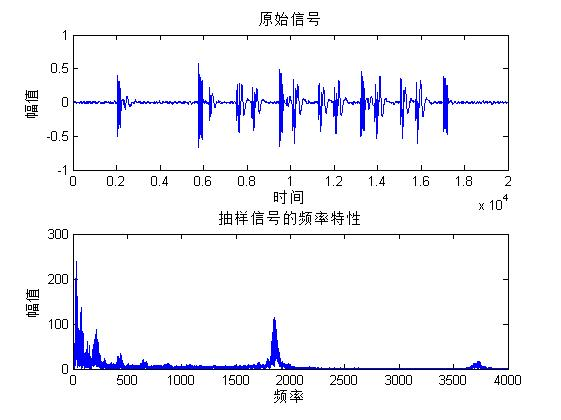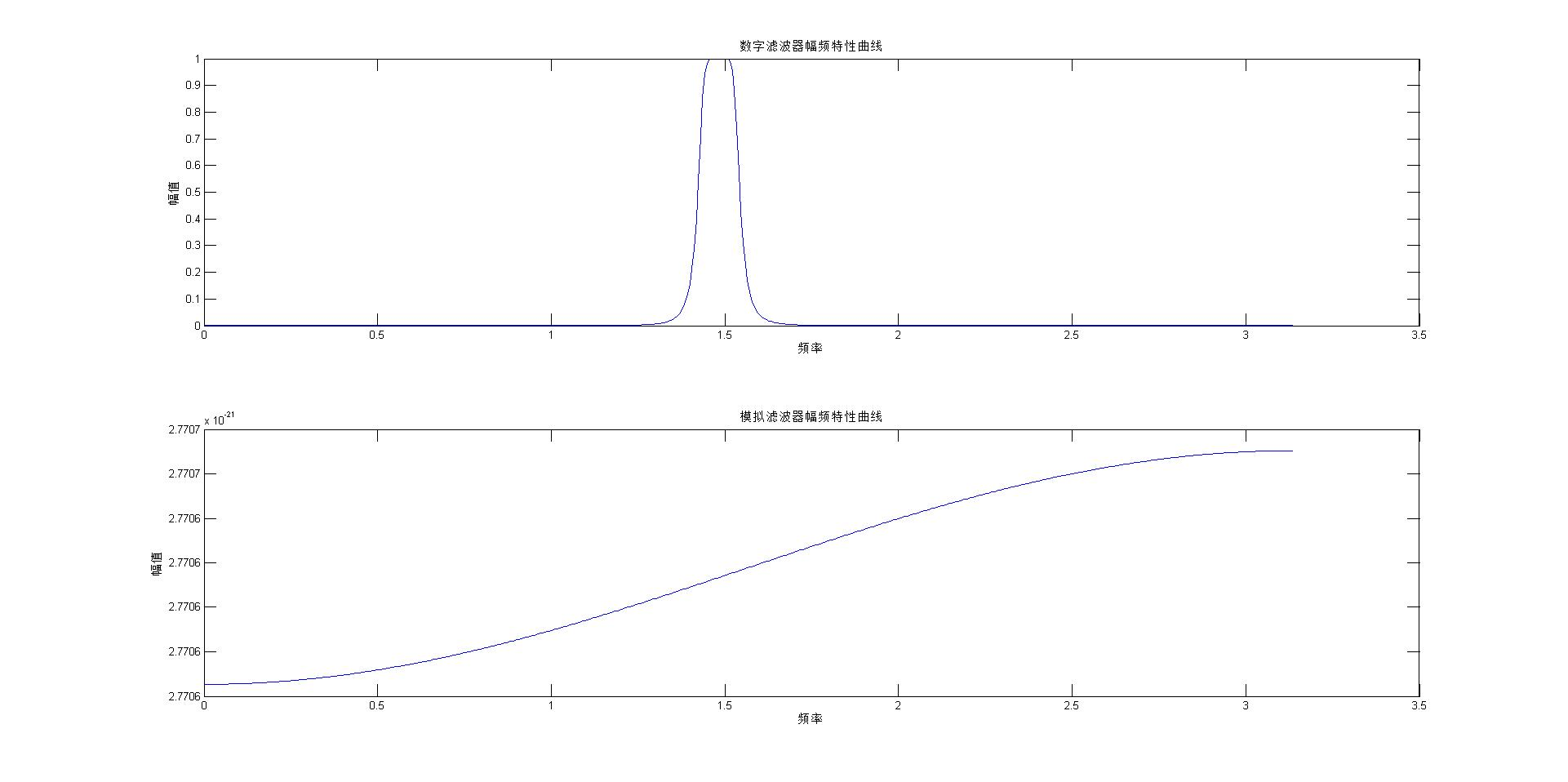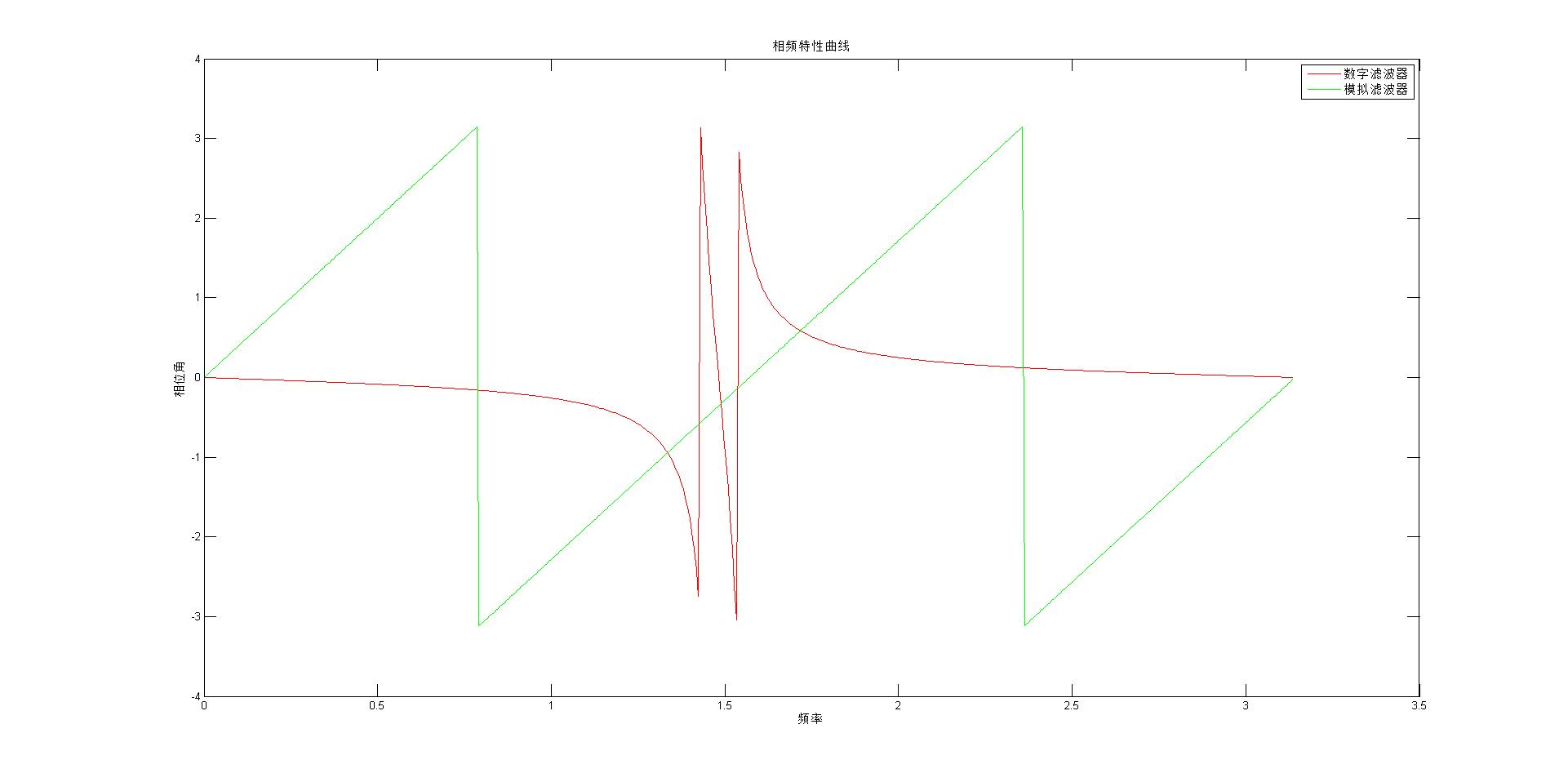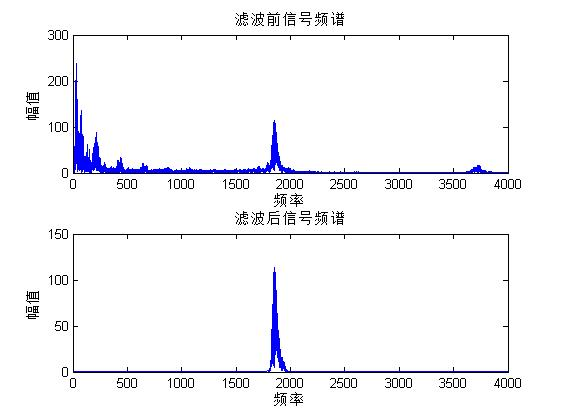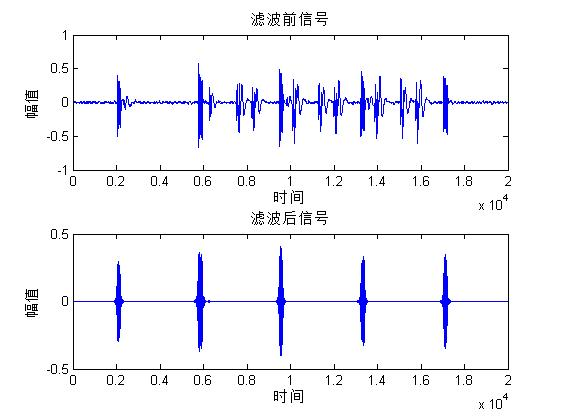源代码
这部分代码直接运行是不可以的，因为还缺少一个数据包heartsignal_part.mat，换成自己的数据就可以运行了。
clc;
clear;
data=data_pata;
len=length(data);
FS=8000; %设置抽样频率

%对信号进行傅里叶变换
fftdata=fft(data);
fftmax=abs(fftdata(1:len/2));%计算幅值
w=(1:len/2)*FS/len;

%绘制原始信号的信息图像
figure(1);
subplot(2,1,1);
plot(data);
title('原始信号');
ylabel('幅值');
xlabel('时间');
subplot(2,1,2);
plot(w,fftmax);
title('抽样信号的频率特性');
ylabel('幅值');
xlabel('频率');

%设置带通滤波器
rp=3;    %通带最大衰减
rs=40;   %阻带最小衰减

%频率转化为角频率
tonglow1=2*pi*1825/FS;   %通带最小
tonghigh1=2*pi*1950/FS;    %通带最大
zulow1=2*pi*1675/FS;     %阻带最小
zuhigh1=2*pi*2100/FS;      %阻带最大

%归一化处理
%预畸变处理，将角频率参数转为模拟滤波器参数
tonglow=2*FS*tan(tonglow1/2);
tonghigh=2*FS*tan(tonghigh1/2);
zulow=2*FS*tan(zulow1/2);
zuhigh=2*FS*tan(zuhigh1/2);

tong=[tonglow tonghigh];
zu=[zulow zuhigh];

[n,Wn]=buttord(tong,zu,rp,rs,'s');
[b,a]=butter(n,Wn,'s');
[bz,az]=bilinear(b,a,FS);  %双线性变换

%滤波器的幅频，相频特性
[H1,h1]=freqz(b,a);
[H,h]=freqz(bz,az);
figure(2);
subplot(2,1,1);
plot(h,abs(H));
title('数字滤波器幅频特性曲线');
ylabel('幅值');
xlabel('频率');
subplot(2,1,2);
plot(h1,abs(H1));
title('模拟滤波器幅频特性曲线');
ylabel('幅值');
xlabel('频率');

figure(9);
plot(h,angle(H),'color','r');
hold on;
plot(h1,angle(H1),'color','g');
title('相频特性曲线');
ylabel('相位角');
xlabel('频率');
legend('数字滤波器','模拟滤波器');

%滤波
outdata=filtfilt(bz,az,data);

%对滤波后信号进行傅里叶变换
fftoutdata=fft(outdata);
fftoutmax=abs(fftoutdata(1:len/2));%计算幅值

figure(3);
subplot(2,1,1);
plot(w,fftmax);
title('滤波前信号频谱');
ylabel('幅值');
xlabel('频率');
subplot(2,1,2);
plot(w,fftoutmax);
title('滤波后信号频谱');
ylabel('幅值');
xlabel('频率');

figure(4);
subplot(2,1,1);
plot(data);
title('滤波前信号');
ylabel('幅值');
xlabel('时间');
subplot(2,1,2);
plot(outdata);
title('滤波后信号');
ylabel('幅值');
xlabel('时间');


展开全文matlab
• 0 前言最近一段时间，在梳理关于滤波器方面的知识。结果，越看越发现自己知识的有限，决定好好补一补；然后找资料的过程中找到一个介绍滤波器，还有其他一些电子信息相关的网站，觉得讲的不错，推荐给大家。1 正文...
• 作为一个电子硬件方面的工作者，怎么能不认识滤波器呢？...时域频域当您在示波器上查看电信号时，您会看到一条线，表示电压随时间的变化。在任何特定时刻，信号只有一个电压值。您在示波器上看到的是信...
• 6. IIR滤波器主要是设计规格化的、频率特性为分段常数的标准低通、高通、带通、带阻、全通滤波器，而FIR滤波器则要灵活得多，例如频率抽样设计法，可适应各种幅度特性的要求，因而FIR滤波器则要灵活得多，例如频率器...
• 最基本的开关电容电路是由电子开关和电容组成的，主要应用是构成各种低通、高通、带通、带阻等开关电容滤波器(Switched-Capacitor Filter，SCF)。将开关电容电路与运算放大器结合，组成的开关电容有源滤波器具有很多...
• 因为做卷积反投影，所以需要推导滤波函数在时域的表达。 R-L 滤波器 HRL(w)={∣w∣∣w∣≤wm0∣w∣>wmH_{RL}(w)=\left\{ \begin{array}{c} |w| && |w|\leq w_m\\ 0 && |w|>w_m \end{array} \...
• 内容简介：课程设计 基于MATLAB的IIR数字带通滤波器设计 共12页，4424字一、数字滤波器数字滤波器是对数字信号实现滤波的线性时不变系统。数字滤波实质上是一种运算过程，实现对信号的运算处理。输入数字信号(数字...
• 1引言随着计算机和信息科学的极大发展,信号处理已经逐步发展成为一门独立...而数字滤波器作为信号处理一项关键技术是数字信号处理的重要基础,在对信号的过滤、检测、与参数估算等处理过程中,它是使用最为广泛的一种...
• 浊音、清音、爆破音的时域频域分析 语音信号具有短时平稳性，因此以下所有的特性分析都基于短时分析技术 语音的时域分析一般有四个重要参数：短时能量、短时过零率、 短时自相关函数 、短时平均幅度差函数 语音的...
• 任何时域的图形，都可以转化为n个不同的频率，振幅和相位的 正选波形 叠加而得到 那么，对一个时域的函数，可以对应到到一个 频率的函数 自变量是 频率 w，因变量是对应这个频率的正弦波形的... 带通滤波器，低通...
• 设计滤波器，对模拟加噪语音信号进行低通滤波、高通滤波及带通滤波，并对滤波前后的信号时域频域进行对比；
• 而对于信号来说，信号强度随时间的变化规律就是时域特性，信号是由哪些单一频率的信号合成的就是频域特性,傅里叶变换实质就是是频域函数和时域函数的转换。 那频域与时域之间的关系是什么样的呢？利用正弦函数的...人工智能 opencv...